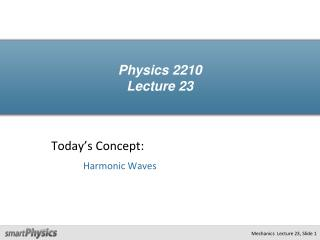DownloadDownload PresentationPhysics 2210 Lecture 23

# Physics 2210 Lecture 23

Télécharger la présentation## Physics 2210 Lecture 23

- - - - - - - - - - - - - - - - - - - - - - - - - - - E N D - - - - - - - - - - - - - - - - - - - - - - - - - - -
##### Presentation Transcript

1. Physics 2210Lecture 23 Today’s Concept: Harmonic Waves

2. A wave is a traveling disturbance that transports energy but not matter. Examples: Sound waves (air moves back & forth) Stadium waves (people move up & down) Water waves (water moves up & down) Light waves (what moves?) What is a Wave?

3. Types of Waves Transverse: The medium oscillates perpendicular to the direction the wave is moving. Longitudinal: The medium oscillates in the same direction as the wave is moving.

4. Wave Properties Amplitude: The maximum displacement Aof a point on the wave. Wavelengthl A Wavelength: The distance λ between identical points on the wave. Period: The time Pit takes for an element of the medium to make one complete oscillation.

5. CheckPoint y A x y B x Waves A and B shown above are propagating in the same medium. How do their frequencies compare? A) ωA>ωB B) ωA<ωB C) ωA= ωB

6. ACT The speed of sound in air is a bit over 300 m/s, and the speed of light in air is about 300,000,000 m/s. Suppose we make a sound wave and a light wave that both have a wavelength of 3 meters. What is the ratio of the frequency of the light wave to that of the sound wave? A) About 1,000,000 B) About 0.000001 C) About 1000

7. Suppose we have some function y = f (x): How to make a Function Move y f(x - a) is just the same shape moveda distance a to the right: x 0 x = a y Let a = vtThen f(x - vt) will describe the same shape moving to the right with speed v. v x 0 x = vt y x 0

8. ACT y = f (x-vt) y y y If a function moving to the right with speed v is described by f (x-vt) then what describes the same function moving to the left with speed v? v x x x 0 0 y A)y = - f (x-vt) v B) y = f (x + vt) x 0 C) y = f (-x + vt)

9. CheckPoint y A x We have shown that the functional form y(x,t) = Acos(kx - ωt) represents a wave moving in the +xdirection. Which of the following represents a wave moving in the –xdirection? A) y(x,t)= Acos(ωt - kx) B) y(x,t)= Asin(kx - ωt) C) y(x,t)= Acos(kx + ωt)

10. Energy

11. CheckPoint y A x y B x Waves A and B shown above are propagating in the same medium with the same amplitude. Which one carries the most energy per unit length? A) A B) B C) They carry the same energy per unit length